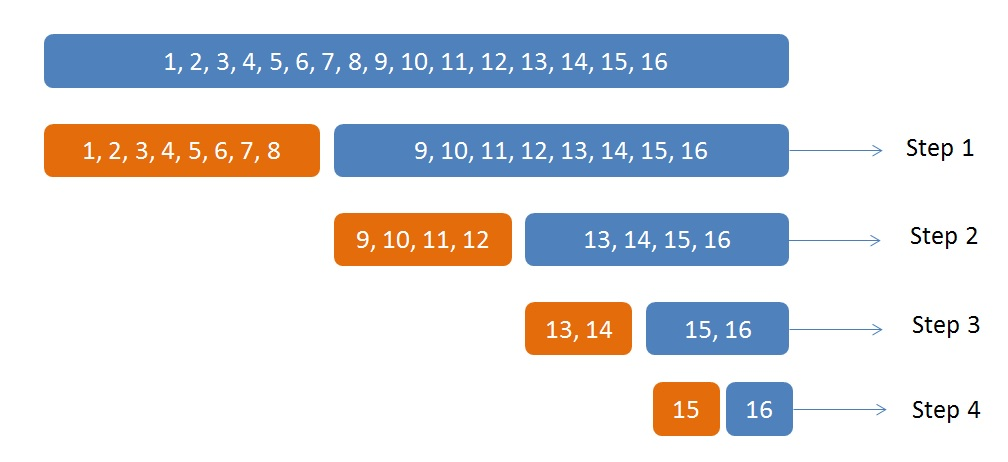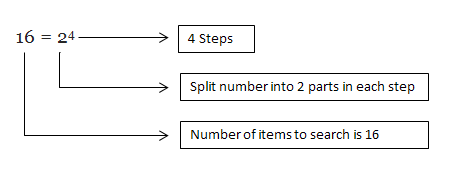{{announcement.body}}
{{announcement.title}}

# Algorithms: Big O Notations Explained

DZone 's Guide to

# Algorithms: Big O Notations Explained

### To represent the efficiency of an algorithm, Big O notations such as O(n), O(1), O(log n) are used. In this article, you'll find examples and explanations of each.

· Big Data Zone ·
Free Resource

Comment (20)

Save
{{ articles.views | formatCount}} Views

This article is intended to explain what Big O notation is in simple terms. Most students and programmers understand O(n) and O(1), but it's a little more difficult to understand O(log n). I have tried my best to explain the three basic Big O notations as simply as possible.

Let's get some background.

## What Is an Algorithm?

Algorithms are methods used to complete a certain operation or solve a problem. We all know that there is more than one way to solve a given problem; similarly, there is more than one algorithm to solve a given problem.

Here's a scenario: If there is more than one algorithm/step to solving a problem, how can we find which is better or more efficient?

In order to represent the efficiency of an algorithm, Big O notations — such as O(n), O(1), O(log n) — are used.

Common Big O notations are:

• O(n): Linear time operation.

• O(1): Constant time operation.

• O(log n): Logarithmic time operation.

In order to understand Big O notation, we need to understand constant time operations, linear time operations, and logarithmic time operations.

Let's now jump into learning these Big O notations one by one with examples/problems.

## O(n): Linear Time Operation

Problem to be solved: Assume that we have a box containing numbers or cards with numbers printed on them (like 1, 2, 3, 4, … 16) and we are asked find if number 6 is in the box. What do we need to do?

Pick one number at a time and check if the number we picked is 6 (the number to search).

If the number we picked matches the number to be searched, then we are good. Otherwise, we need to pick another number from the box. This way of picking one number at a time and verifying if it matches one after another until all the n numbers are picked is called linear operation. This way of searching n numbers is represented as O(n). If the number to find is the last number (the worst case scenario), we need to pick all the n numbers.

``````int n = 16;
int[] numbers = {11,12,13,14,15,16,1,2,3,4,5,6,7,8,9,10};
int numberToSearch = 16;

int find(int numberToSearch, int[] numbers) {
for (int i = 0; i < n; i++) {
if (numbers[i] = numberToSearch)
return i; //FOUND
}
}``````

## O(1): Constant Time Operation

Problem to be solved: Assume we are given a box of numbers (1, 2, 3, 4, … 16) and outside the box it's printed that the box contains 16 numbers. You are asked how many numbers/items are there in the box. Because you know the box is labeled as containing 16 balls, you reply saying the box contains 16. If another person asks you the same question next day, you can answer again just by looking at the box, even if you get another box with 100 numbers in it and if it has a label saying the box contains 100 numbers. This is called constant time operation. It is represented as O(1).

``````int n = 16;
int[] numbers = {11,12,13,14,15,16,1,2,3,4,5,6,7,8,9,10};

int findSize(int[] numbers) {
return numbers.length;
}``````

### O(log n): Logarithmic Time Operation

Problem to be solved: Assume we have a box that contains numbers (1, 2, 3, 4, … 16) and all the numbers are in order. You are asked to find a number 16 in the box. The catch here is that the numbers are in order. Let's split the numbers into two parts. A total of 16 numbers is divided into two sets each containing eight numbers.

``````int n = 16;
int[] numbers = {1,2,3,4,5,6,7,8,9,10,11,12,13,14,15,16};
int numberToSearch = 16;

int median = 16/2 = 8;
int[] split1 = {1,2,3,4,5,6,7,8};
int[] split2 = {9,10,11,12,13,14,15,16};``````

Number 16 is greater than the greatest element in the split, hence the number to search will only be split in 2. Continue this splitting until the end of numbers or the number to search is found.

Thus:Looking at the above picture, we are able to find a number in4 steps (in a list of 16 numbers).

This can be written as,In math, if n = 2xthen log2 n = x. (Refer Binary Logarithm)

Hence 16 = 24 can be written as log2 16 = 4.

This can be written as logn, or simply O(log n).

Thus, four steps are required to find a number in a box containing 16 numbers splitting the box into two every step (this is also called binary search).

``````int find(int[] numbers, int numberToSearch) {
int left = 0;
int right = numbers.length - 1;

while (left <= right) {
int mid = left + (right - left) / 2;

if (numberToSearch < numbers[mid]){
right = mid - 1;
}
else if (numberToSearch > numbers[mid]) {
left = mid + 1;
}
else {
return mid; //NUMBER FOUND
}
}
}``````

I hope this is simple or at least easy to understand!

Another example:

If for example, we have 64 numbers, then maximum number of steps to find a number can be derived as below,

64 = 26, this can be written as log64 = 6. Hence maximum of 6 steps is required to find a number in a list of 64 numbers.

Topics:
algorithms ,big o ,tutorial

Comment (20)

Save
{{ articles.views | formatCount}} Views

Opinions expressed by DZone contributors are their own.